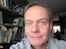# Math Is Fun Forum

Discussion about math, puzzles, games and fun.   Useful symbols: ÷ × ½ √ ∞ ≠ ≤ ≥ ≈ ⇒ ± ∈ Δ θ ∴ ∑ ∫  π  -¹ ² ³ °

You are not logged in.

## #1 2023-09-10 23:11:44

Ivar Sand
MemberRegistered: 2013-01-22
Posts: 16

### Proof that the standard procedure for adding two numbers is correct

Example of adding two numbers a and b with the result s:

c    101
a     404
b     678
s    1082

Here, c stands for "carry"(2).

The general schema for adding two non-negative integers(1) a and b looks like this:

where n is a natural number(1).
Note that the sequence(5) of digits(3) for c (the carry) is placed one position to the left with respect to the sequences of digits for a and b.

Below, we shall benefit from the definition of x modulo 10(4):
x mod 10 = x1
and from the definition of x // 10:
x // 10 = (x - x1) / 10
where x stands for a non-negative integer(1) with x1 as its least significant digit.
We note that:
x mod 10 picks out the least significant digit of x
x // 10 shifts the digits of x one position to the right and discards the digit to the right of the decimal point

Let n be a natural number(1).

Let the non-negative integers(1) c, a, b, and s be represented by their sequences(5) of digits(3):

so that the values of a, b, and s can be expressed, respectively:

The number of digits is set to n for both a and b.
If one of a and b should at the outset have less than n number of digits, its number of digits is increased by extending its sequence of digits to the left with digits equal to 0 until its number of digits equals n.

According to the general schema for adding two non-negative integers, we define the digits of c like this:

and we define the digits of s like this:

Then:

in other words:

We take a closer look at s:

as stated in the theorem.

Let n be a natural number(1).
Let x be a non-negative integer(1) represented by its sequence(5) of digits(3):

Let:

Let:

Then:

We have:

as stated in the lemma.

References:
(1) Natural number (non-negative integers) https://en.wikipedia.org/wiki/Natural_number
(2) Carry (arithmetic) https://en.wikipedia.org/wiki/Carry_(arithmetic)
(3) Numerical digit https://en.wikipedia.org/wiki/Numerical_digit
(4) Modulo Operation https://www.mathsisfun.com/numbers/modulo.html
(5) Sequences https://www.mathsisfun.com/algebra/sequences-series.html

I majored in Physics in 1976. Also, I studied mathematics and computer science. I work as a computer programmer. I am from Norway.

Offline